# Seaborn Heatmap – A comprehensive guide

• Difficulty Level : Medium
• Last Updated : 12 Nov, 2020

Heatmap is defined as a graphical representation of data using colors to visualize the value of the matrix. In this, to represent more common values or higher activities brighter colors basically reddish colors are used and to represent less common or activity values, darker colors are preferred. Heatmap is also defined by the name of the shading matrix. Heatmaps in Seaborn can be plotted by using the seaborn.heatmap() function.

### seaborn.heatmap()

Syntax: seaborn.heatmap(data, *, vmin=None, vmax=None, cmap=None, center=None, annot_kws=None, linewidths=0, linecolor=’white’, cbar=True, **kwargs)

Important Parameters:

• data: 2D dataset that can be coerced into an ndarray.
• vmin, vmax: Values to anchor the colormap, otherwise they are inferred from the data and other keyword arguments.
• cmap: The mapping from data values to color space.
• center: The value at which to center the colormap when plotting divergent data.
• annot: If True, write the data value in each cell.
• fmt: String formatting code to use when adding annotations.
• linewidths: Width of the lines that will divide each cell.
• linecolor: Color of the lines that will divide each cell.
• cbar: Whether to draw a colorbar.

All the parameters except data are optional.

Returns: An object of type matplotlib.axes._subplots.AxesSubplot

Let us understand the heatmap with examples.

### Basic Heatmap

Making a heatmap with the default parameters. We will be creating a 10×10 2-D data using the randint() function of the NumPy module.

## Python3

 `# importing the modules``import` `numpy as np``import` `seaborn as sn``import` `matplotlib.pyplot as plt`` ` `# generating 2-D 10x10 matrix of random numbers``# from 1 to 100``data ``=` `np.random.randint(low ``=` `1``,``                         ``high ``=` `100``,``                         ``size ``=` `(``10``, ``10``))``print``(``"The data to be plotted:\n"``)``print``(data)`` ` `# plotting the heatmap``hm ``=` `sn.heatmap(data ``=` `data)`` ` `# displaying the plotted heatmap``plt.show()`

Output:

```The data to be plotted:

[[46 30 55 86 42 94 31 56 21  7]
[68 42 95 28 93 13 90 27 14 65]
[73 84 92 66 16 15 57 36 46 84]
[ 7 11 41 37  8 41 96 53 51 72]
[52 64  1 80 33 30 91 80 28 88]
[19 93 64 23 72 15 39 35 62  3]
[51 45 51 17 83 37 81 31 62 10]
[ 9 28 30 47 73 96 10 43 30  2]
[74 28 34 26  2 70 82 53 97 96]
[86 13 60 51 95 26 22 29 14 29]]
```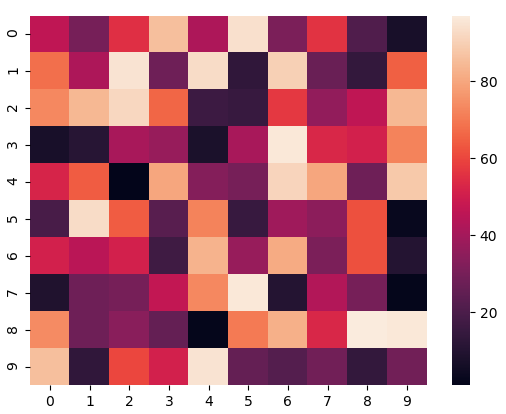We’ll be using this same data in all the examples.

### Anchoring the colormap

If we set the vmin value to 30 and the vmax value to 70, then only the cells with values between 30 and 70 will be displayed. This is called anchoring the colormap.

## Python3

 `# importing the modules``import` `numpy as np``import` `seaborn as sn``import` `matplotlib.pyplot as plt`` ` `# generating 2-D 10x10 matrix of random numbers``# from 1 to 100``data ``=` `np.random.randint(low``=``1``,``                         ``high``=``100``,``                         ``size``=``(``10``, ``10``))`` ` `# setting the parameter values``vmin ``=` `30``vmax ``=` `70`` ` `# plotting the heatmap``hm ``=` `sn.heatmap(data``=``data,``                ``vmin``=``vmin,``                ``vmax``=``vmax)`` ` `# displaying the plotted heatmap``plt.show()`

Output: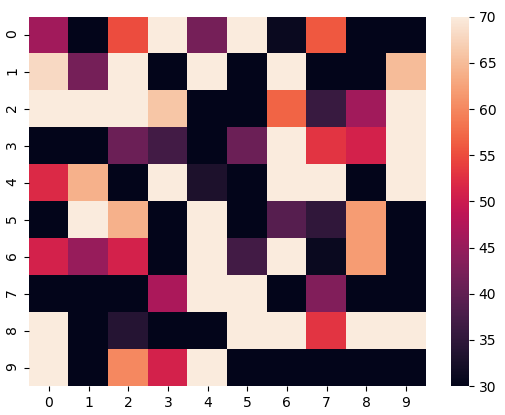### Choosing the colormap

In this, we will be looking at the cmap parameter. Matplotlib provides us with multiple colormaps, you can look at all of them here. In our example, we’ll be using tab20.

## Python3

 `# importing the modules``import` `numpy as np``import` `seaborn as sn``import` `matplotlib.pyplot as plt`` ` `# generating 2-D 10x10 matrix of random numbers``# from 1 to 100``data ``=` `np.random.randint(low``=``1``,``                         ``high``=``100``,``                         ``size``=``(``10``, ``10``))`` ` `# setting the parameter values``cmap ``=` `"tab20"`` ` `# plotting the heatmap``hm ``=` `sn.heatmap(data``=``data,``                ``cmap``=``cmap)`` ` `# displaying the plotted heatmap``plt.show()`

Output:### Centering the colormap

Centering the cmap to 0 by passing the center parameter as 0.

## Python3

 `# importing the modules``import` `numpy as np``import` `seaborn as sn``import` `matplotlib.pyplot as plt`` ` `# generating 2-D 10x10 matrix of random numbers``# from 1 to 100``data ``=` `np.random.randint(low``=``1``,``                         ``high``=``100``,``                         ``size``=``(``10``, ``10``))`` ` `# setting the parameter values``cmap ``=` `"tab20"``center ``=` `0`` ` `# plotting the heatmap``hm ``=` `sn.heatmap(data``=``data,``                ``cmap``=``cmap,``                ``center``=``center)`` ` `# displaying the plotted heatmap``plt.show()`

Output: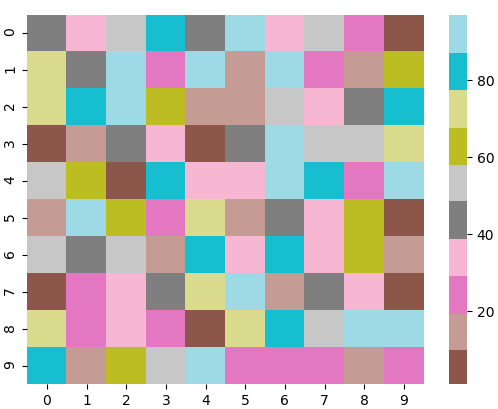### Displaying the cell values

If we want to display the value of the cells, then we pass the parameter annot as True. fmt is used to select the datatype of the contents of the cells displayed.

## Python3

 `# importing the modules``import` `numpy as np``import` `seaborn as sn``import` `matplotlib.pyplot as plt`` ` `# generating 2-D 10x10 matrix of random numbers``# from 1 to 100``data ``=` `np.random.randint(low``=``1``,``                         ``high``=``100``,``                         ``size``=``(``10``, ``10``))`` ` `# setting the parameter values``annot ``=` `True`` ` `# plotting the heatmap``hm ``=` `sn.heatmap(data``=``data,``                ``annot``=``annot)`` ` `# displaying the plotted heatmap``plt.show()`

Output: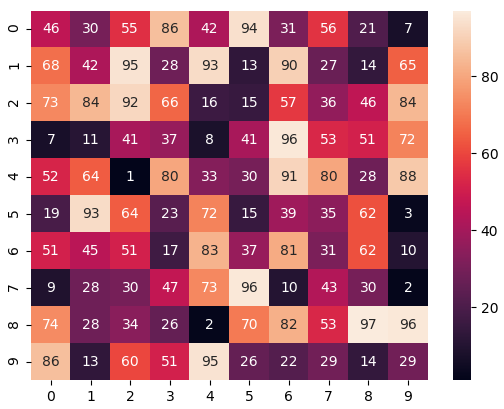### Customizing the separating line

We can change the thickness and the color of the lines separating the cells using the linewidths and linecolor parameters respectively.

## Python3

 `# importing the modules``import` `numpy as np``import` `seaborn as sn``import` `matplotlib.pyplot as plt`` ` `# generating 2-D 10x10 matrix of random numbers``# from 1 to 100``data ``=` `np.random.randint(low``=``1``,``                         ``high``=``100``,``                         ``size``=``(``10``, ``10``))`` ` `# setting the parameter values``linewidths ``=` `2``linecolor ``=` `"yellow"`` ` `# plotting the heatmap``hm ``=` `sn.heatmap(data``=``data,``                ``linewidths``=``linewidths,``                ``linecolor``=``linecolor)`` ` `# displaying the plotted heatmap``plt.show()`

Output: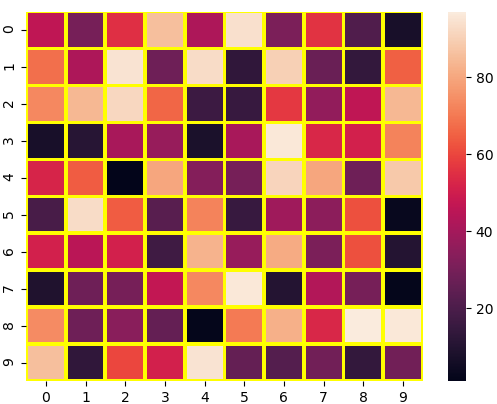### Hiding the colorbar

We can disable the colorbar by setting the cbar parameter to False.

## Python3

 `# importing the modules``import` `numpy as np``import` `seaborn as sn``import` `matplotlib.pyplot as plt`` ` `# generating 2-D 10x10 matrix of random numbers``# from 1 to 100``data ``=` `np.random.randint(low``=``1``,``                         ``high``=``100``,``                         ``size``=``(``10``, ``10``))`` ` `# setting the parameter values``cbar ``=` `False`` ` `# plotting the heatmap``hm ``=` `sn.heatmap(data``=``data,``                ``cbar``=``cbar)`` ` `# displaying the plotted heatmap``plt.show()`

Output: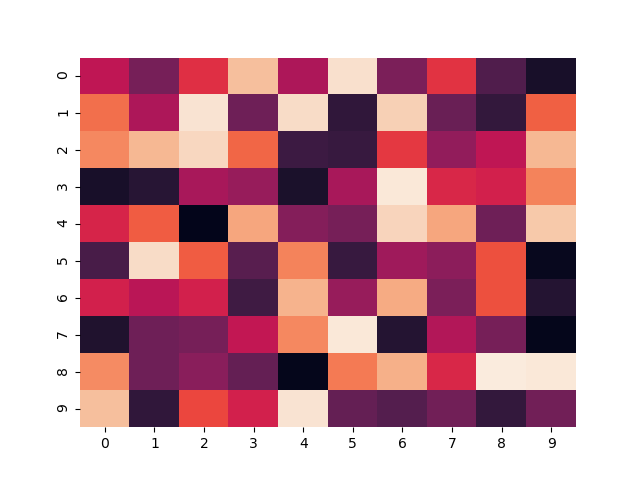### Removing the labels

We can disable the x-label and the y-label by passing False in the xticklabels and yticklabels parameters respectively.

## Python3

 `# importing the modules``import` `numpy as np``import` `seaborn as sn``import` `matplotlib.pyplot as plt`` ` `# generating 2-D 10x10 matrix of random numbers``# from 1 to 100``data ``=` `np.random.randint(low``=``1``,``                         ``high``=``100``,``                         ``size``=``(``10``, ``10``))`` ` `# setting the parameter values``xticklabels ``=` `False``yticklabels ``=` `False`` ` `# plotting the heatmap``hm ``=` `sn.heatmap(data``=``data,``                ``xticklabels``=``xticklabels,``                ``yticklabels``=``yticklabels)`` ` `# displaying the plotted heatmap``plt.show()`

Output:My Personal Notes arrow_drop_up